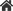Home > News Information > Industry News > Numerical analysis of air-cooled straight finned tube heat exchanger

## Numerical analysis of air-cooled straight finned tube heat exchanger

2021-12-02Industry News1400
```Numerical analysis of air-cooled straight finned tube heat exchanger Finned tube heat exchanger is widely used in refrigeration
and air conditioning systems. As one of the key components, the performance and efficiency of heat exchanger is very important
to the whole system. With the development of computational heat transfer, using numerical methods to study the flow and heat
transfer inside the heat exchanger has become a research hotspot in recent years. This method has the advantages of low cost and
can simulate more complex processes. In this paper, numerical study on air-cooled straight finned tube condenser is carried out.```

1. Physical problems The research object of this paper is the integral straight finned tube commonly used in air conditioning equipment, and its geometric dimensions are shown in Table 1. Due to the periodicity and symmetry of integral finned tube heat exchanger, we can choose a calculation unit to study. In addition, in order to avoid the influence of backflow at the outlet boundary on the calculation results, the calculation area is extended by 10 times to ensure that there is no backflow at the outlet boundary. A hexahedral grid is generated for the selected control volume, and the total number of points is 160X12X10 (excluding the extended area).
2. The governing equations are used to calculate the three-dimensional flow, assuming that the air flow is incompressible, laminar and steady. The physical parameters of air are constant.
3. Boundary condition The tube wall is a solid boundary with a constant temperature of 308.15K (35 ℃); The upper and lower fins are solid boundary, and the constant wall temperature is 308.15K (35 ℃); The incoming air temperature is 298.15K (25 ℃). Since the air temperature changes little, its physical parameters are taken as constants (parameters at 300K). The outflow boundary is partially unidirectional, and the other boundaries are symmetric boundary conditions.

```4. Analysis and discussion of the results Fig.1 shows the temperature distribution on the central section of the control volume when the incoming air velocity y = 2.0m / s. It can be
clearly seen from the figure that the wake area behind the two rows of tubes has backflow, and the temperature is significantly higher than the surrounding area. Fig.2 shows the pressure
distribution on the central section, and the pressure change trend can be seen. The pressure change between the two rows of pipes is small. This is determined by two reasons : on the one
hand, the decrease of flow rate will increase the static pressure, and on the other hand, the shear force will reduce the pressure. There is some pressure oscillation between the two rows
of tubes, which is caused by the change of local air velocity. In the case of other different incoming air flow velocities calculated in this paper, the temperature and pressure change trends
are the same.```
```5.ConclusionIn this paper, the numerical calculation of the air-cooled condenser with two rows of integral flat fins at different air inflow velocities is carried out, and the temperature field
and pressure field distribution on the air side are obtained. The more complex situation remains to be further studied.```